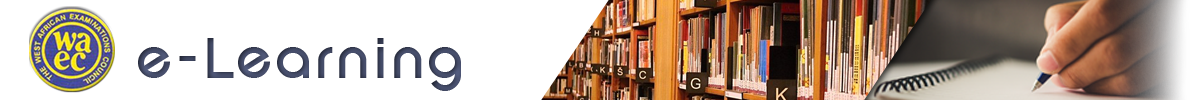Physics (Essay) Paper 2 WASSCE (SC), 2016

Question 10

a) Define critical angle

1. (b) How are anti-nodes created in a stationary wave?
2. (c) The angle of minimum deviation of an equilateral triangular glass prism is 46.20. Calculate the refractive index of the glass.
3. (d) An illuminated object is placed in front of a concave mirror and the position of a screen is adjusted in front of the mirror but no image is obtained on the screen. Give two possible reasons for this observation.
4. (e) An illuminated object is placed at a distance of 75 cm from a converging lens of focal length 30 cm.
5. i. Determine the image distance
6. ii. If the lens is replaced by another converging lens, the object has to be moved 25 cm further away to have its sharp image on the screen. Determine the focal length of the second lens.

Observation

Part (a):     Performance was poor as many candidates could not give the correct definition of critical angle. Some candidates lost the mark due to failure to state that “motion was from denser to less dense medium”

Part (b):     Performance was poor as many candidates could not explain how antinodes are created in a stationary wave.

Part (c):     Performance was good. However, many candidates did not state the formula properly and therefore the substitution was wrong to arrive at a wrong answer. Also, many candidates skipped this question.

Part (d):     Candidates performed averagely. More than 50% of the candidates did not get the two reasons right.

Part (e) (i): Performance was good because many candidates used the correct formula to substitute to solve the problem.

(ii):       A few candidates were able to obtain the new value of u and substitute to get the new value of f.